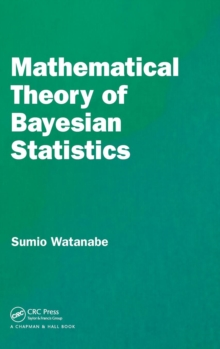# Mathematical Theory of Bayesian Statistics Hardback

#### Description

Mathematical Theory of Bayesian Statistics introduces the mathematical foundation of Bayesian inference which is well-known to be more accurate in many real-world problems than the maximum likelihood method.

Recent research has uncovered several mathematical laws in Bayesian statistics, by which both the generalization loss and the marginal likelihood are estimated even if the posterior distribution cannot be approximated by any normal distribution.

FeaturesExplains Bayesian inference not subjectively but objectively.

Provides a mathematical framework for conventional Bayesian theorems. Introduces and proves new theorems. Cross validation and information criteria of Bayesian statistics are studied from the mathematical point of view.

Illustrates applications to several statistical problems, for example, model selection, hyperparameter optimization, and hypothesis tests.

This book provides basic introductions for students, researchers, and users of Bayesian statistics, as well as applied mathematicians. AuthorSumio Watanabe is a professor of Department of Mathematical and Computing Science at Tokyo Institute of Technology.

He studies the relationship between algebraic geometry and mathematical statistics.

#### Information

• Format: Hardback
• Pages: 320 pages, 50 Illustrations, black and white
• Publisher: Apple Academic Press Inc.
• Publication Date:
• ISBN: 9781482238068

£140.00

£112.03

Free delivery within the UK£37.49

£33.74

Available for